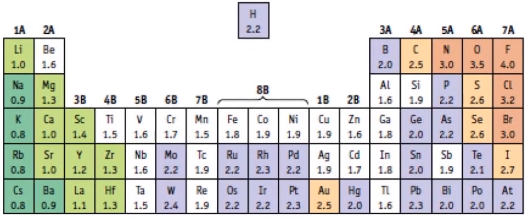# Problem: Which of the bonds between the following pairs of atoms would be the least polar?A) Na, SB) P, SC) C, FD) Si, ClE) Na, Cl

###### FREE Expert Solution

Determine which among the given pair of atoms is the least polar by calculating the electronegative difference of each atom

Recall that the lower the electronegativity difference, the less polar a bond is. Using an electronegativity chart, the EN difference will appear as:###### Problem Details

Which of the bonds between the following pairs of atoms would be the least polar?

A) Na, S

B) P, S

C) C, F

D) Si, Cl

E) Na, Cl